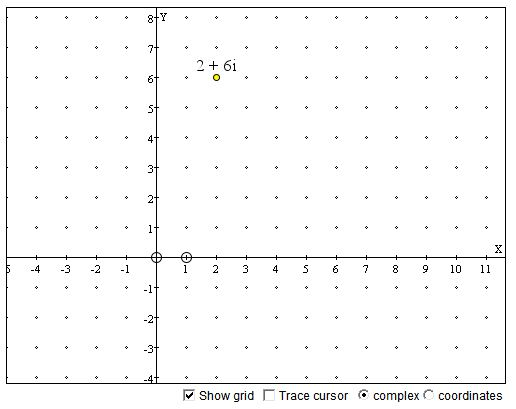# Cartesian Coordinate System

A straight line with an associated direction, a selected point and a unit length is known as the number line, especially when the numbers of interest are integers. Otherwise, it may be called a number or real axis. The selected point is called the origin. Points on the real axis relate to real numbers such that the origin is associated with $0$ and the point at the unit distance from it in the chosen direction with $1.$ All other points are assigned real numbers which are their distances to the origin measured with the given unit length and taken with the sign plus or minus depending whether they are on the same side from the origin as the number $1.$

(It is assumed that any point on a line divides the line into two rays so that the division point separates the points on the rays. In case of a real axis and its origin, the rays are known as the positive and negative half-axes).

The number associated with a point is called its coordinate.

Two perpendicular real axes in the plane define a (rectangular planar) Cartesian coordinate system. Their common point is taken to be the origin (for both of them) and the two unit lengths are commonly equal. Usually, but not always, one of the two axes is horizontal, the other vertical; their positive directions are to the right and upwards. Usually, but again not always, the horizontal axis is called $x$-axis, the vertical one is called $y$-axis.

With a Cartesian system in place, any point in the plane is associated with an ordered pair of real numbers. To obtain these number, we draw to lines through the point parallel (and hence perpendicular) to the axes. We are interested in the coordinates of the points of intersection of the two lines with the axes. Assuming the given point does not lie on either of the axes, there are two cooridnates: $x$-coordinate on the $x$-axis and $y$-coordinate on the $y$-axis. The $x$-coordinate is called the absissa and the $y$-coordinate is called the ordinate of the point at hand. These are the two numbers associated with the point. They are usually written as $(x, y),$ the absissa coming first, the ordinate second.

Complex numbers are points in the plane endowed with additional structure. The $y$-unit is then denoted $i$ and the points on the $y$-axis are written as $yi.$ The points on the $x$-axis are denoted by the single real number $x,$ as if it was the only number axis. Instead of $(x, y),$ we then write $x + yi$ and call the expression the complex coordinate of a point. Thus

$x + yi = (x, y).$

The sign "$+$" really signifies an operation of addition defined for complex numbers. In fact, $yi$ actually means the product of $y$ and $i.$ The two claims imply $yi = 0 + yi$ and $x + 0i = x.$

### If you are reading this, your browser is not set to run Java applets. Try IE11 or Safari and declare the site https:///www.cut-the-knot.org as trusted in the Java setup.What if applet does not run?

For a point $(x, y)$ the distance to the origin $(0, 0)$ is determined from the Pythagorean theorem,

 (1) $dist((0,0), (x,y)) = \sqrt{x^{2}+y^{2}}.$

More generally, the distance between two points $(x_{1}, y_{1})$ and $(x_{2}, y_{2})$ is defined as

 (2) $dist((x_{1},y_{1}), (x_{2},y_{2})) = \sqrt{(x_{1}-x_{2})^{2}+(y_{1}-y_{2})^{2}}.$

In complex notations, we write instead

 (1') $|x+yi| = \sqrt{x^{2}+y^{2}}.$

and call $|x + yi|$ the modulus, or absolute value, of the complex number $x + yi.$### Complex Numbers• What Is Line?
• Functions, what are they?
• Addition and Subtraction of Functions
• Function, Derivative and Integral
• Graph of a Polynomial of arbitrary degree
• Graph of a Polynomial Defined by Its Roots
• Inflection Points of Fourth Degree Polynomials
• Lagrange Interpolation (an Interactive Gizmo)
• Equations of a Straight Line
• Taylor Series Approximation to Cosine
• Taylor Series Approximation to Cosine
• Linear Function with Coefficients in Arithmetic Progression
• Sine And Cosine Are Continuous Functions
•